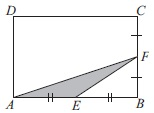# User Forum

Subject :IMO    Class : Class 7

ABCD is a rectangle. E and F are midpoints of AB and CB respectively. If the area of the shaded ΔAEF is 7 cm2, then the area of rectangle ABCD, is____ .A28 cm2
B49 cm2
C35 cm2
D56 cm2

Class : Class 9

Class : Class 9

Class : Class 8

Class : Class 9
How do we do it

## Ans 5:

Class : Class 7
area of triangle = 1â2 Ã (Base) Ã (Height)= 1â2 Ã (2x) Ã (y)(1) (2) = 1â2 Ã (2x) Ã (y) = xy(1) = 1â2 xyxyâ2 7 = xyxy = 14Area of ABCD = (2x) Ã (2y) = 4xy4 Ã 14 = 56 cm2